# Analysing time-series of Quandl

### 2013/11/16 02:37:50 PM

Analysing S&P 500 Index downloaded from Quandl in 6.76 seconds with the following original description:

GSPC: S&P 500 Index

## Variables

This daily dataset contains 16073 rows and 7 columns with the overall number of 112511 records, from which 32146 records will be analysed for the Open variable.

# Overview

The descriptive statistics of Open can be used to provide a quick and dirty overview of the data:

min mean median max sd IQR
16.7 434 129 1791 493 769

## Histogram

The below histogram also shows that the values are somewhere between 16.7 and 1791 (range: 1774) with the average mean being 434: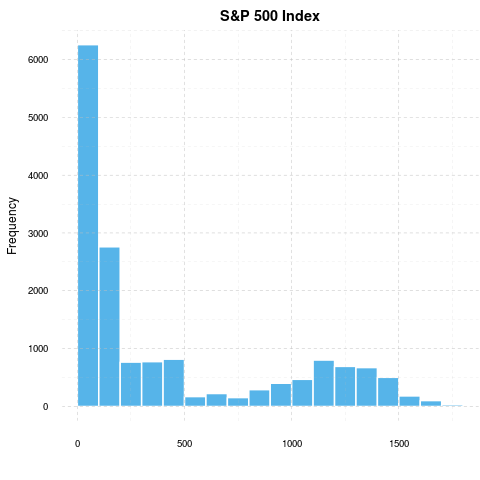Histogram of Open

If the data is not normal, it is also worth checking out the median (129) and the interquartile range (769) too instead of the standard deviation (493).

References:

• Becker, R. A., Chambers, J. M. and Wilks, A. R. (1988) The New S Language. Wadsworth & Brooks/Cole.
• Chambers, J. M. and Hastie, T. J. (1992) Statistical Models in S. Wadsworth & Brooks/Cole.
• Venables, W. N. and Ripley. B. D. (2002) Modern Applied Statistics with S. Springer.

# Observed values

The above histogram shows not much about a time-series, right? Let us check out other options.

## Line plot

The daily data between 1950-01-03 and 2013-11-15 on a line-plot: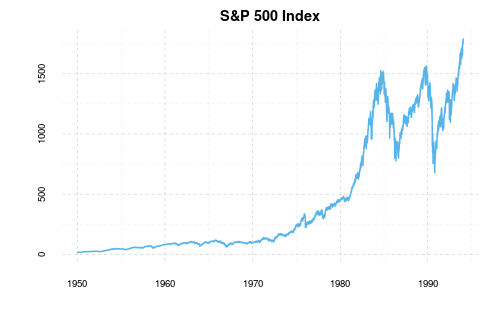## Heatmap

Which looks much better on a calendar heatmap: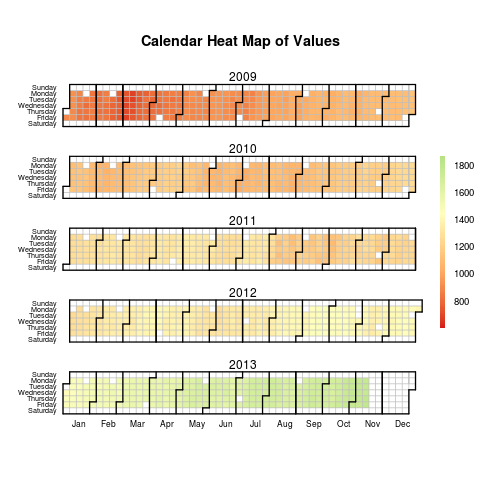Please note that only the last 5 years were shown above. Please register at rapporter.net for dedicated resources.

References:

• Paul Bleicher (2009) calendarHeat R function
• Jason Bryer (2012) makeR: Package for managing projects with multiple versions derived from a single source repository. R package version 1.0.2. http://CRAN.R-project.org/package=makeR

# Autocorrelation

Computing the cross-correlation of a signal with itself is a mathematical tool for finding repeating patterns in the time-series. Basically we compute the correlation coefficient between the raw data and its lagged version for serveral iterations, where high (>0.5) or low (<-0.5) values show a repeating pattern.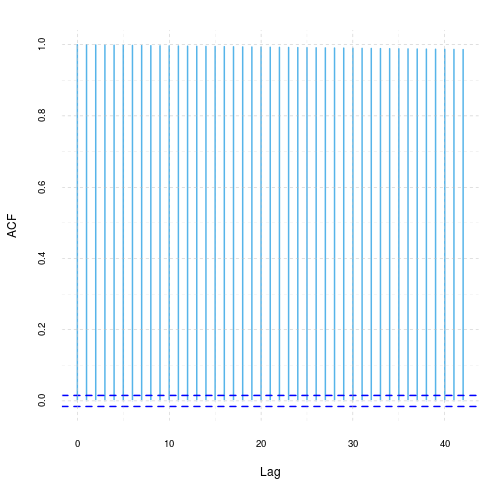The autocorrelation estimate is maximum at lag 1 being 1.

References:

• Venables, W. N. and Ripley, B. D. (2002) Modern Applied Statistics with S. Fourth Edition. Springer-Verlag.

# Seasonal effects

Computing a quick and dirty seasonal-effect with the frequency being 365: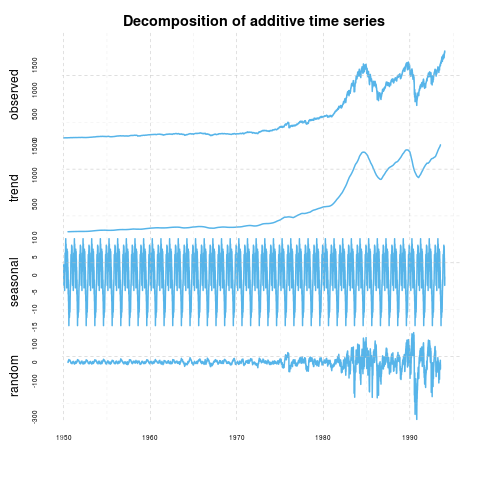Where the seasonal effect for a period looks like: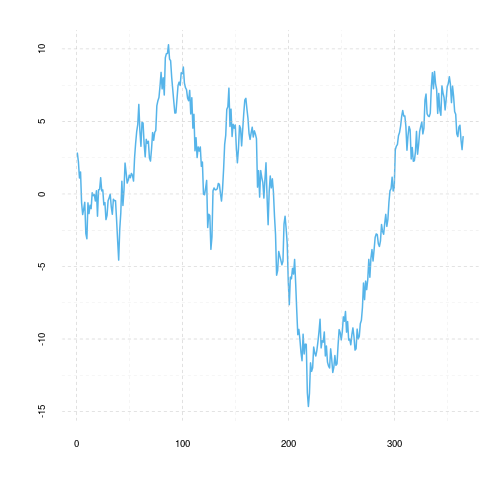References:

• M. Kendall and A. Stuart (1983) The Advanced Theory of Statistics, Vol.3, Griffin. pp. 410-414.

# Linear model

And now we build a really simple linear model based on the year, the month, the day of the month and also the day of the week to predict S&P 500 Index. The model that can be built automatically is: value ~ year + month + mday + wday.

## Assumptions

In order to have reliable results, we have to check if the assumptions of the linear regression met with the data we used:

Value p-value Decision
Global Stat 11368 0 Assumptions NOT satisfied!
Skewness 256 0 Assumptions NOT satisfied!
Kurtosis 573 0 Assumptions NOT satisfied!
Link Function 9589 0 Assumptions NOT satisfied!
Heteroscedasticity 951 0 Assumptions NOT satisfied!

To check these assumptions, the Global Validation of Linear Model Assumptions R-package will help us. The result of that we can see in the table above.

The GVLMA makes a thorough detection on the linear model, including tests generally about the fit, the shape of the distribution of the residuals (skewness and kurtosis), the linearity and the homoskedasticity. On the table we can see if our model met with the assumptions. As a generally accepted thumb-rule we use the critical p-value=0.05.

So let's see the results, which the test gave us:

• The general statistic tells us about the linear model, that it does not fit to our data.

• According to the GVLMA the residuals of our model's skewness differs significantly from the normal distribution's skewness.

• The residuals of our model's kurtosis differs significantly from the normal distribution's kurtosis, based on the result of the GVLMA.

• In the row of the link function we can read that the linearity assumption of our model was rejected.

• At last but not least GVLMA confirms the violation of homoscedasticity.

In summary: We can 't be sure that the linear model used here fits to the data.

References:

• Pena, EA and Slate, EH (2006): Global validation of linear model assumptions. J. Amer. Statist. Assoc. 101 (473):341-354.

## Linearity

As we want to fit a linear regression model, it is advisable to see if the relationship between the used variables are linear indeed. Next to the test statistic of the GVLMA it is advisable to use a graphical device as well to check that linearity. Here we will use the so-called crPlots funtion to do that, which is an abbreviation of the Component and Residual Plot.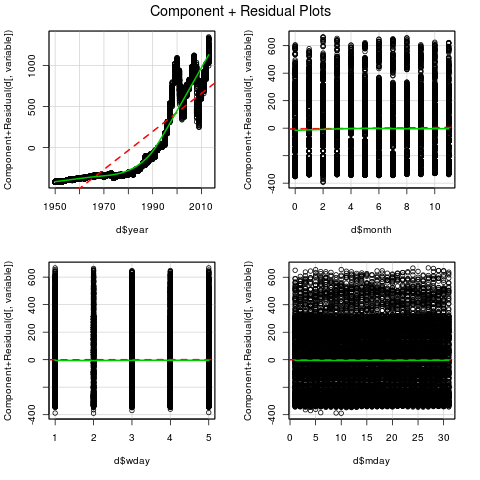First, we can see two lines and several circles. The red interrupted line is the best fitted linear line, which means that te square of the residuals are the least while fitting that line in the model. The green curved line is the best fitted line, which does not have to be straight, of all. The observations we investigate are the circles. We can talk about linearity if the green line did not lie too far from the red.

References:

• Cook, R. D. and Weisberg, S. (1999) Applied Regression, Including Computing and Graphics. Wiley.
• Fox, J. (2008) Applied Regression Analysis and Generalized Linear Models, Second Edition. Sage.
• Fox, J. and Weisberg, S. (2011) An R Companion to Applied Regression, Second Edition, Sage.

## Parameters

A linear model: value ~ year + month + mday + wday
Estimate Std. Error t value Pr(>|t|)
d$year 23.2 0.105 221 0 d$month 1.04 0.565 1.84 0.0663
d$wday 0.285 1.38 0.207 0.836 d$mday 0.0286 0.222 0.129 0.897
(Intercept) -45507 208 -218 0

Most model parameters can be read from the above table, but nothing about the goodness of fit. Well, the R-squared turned out to be 0.752 while the adjusted version is 0.752.

References:

• Chambers, J. M. (1992) Linear models. Chapter 4 of Statistical Models in S eds J. M. Chambers and T. J. Hastie, Wadsworth & Brooks/Cole.

• Wilkinson, G. N. and Rogers, C. E. (1973) Symbolic descriptions of factorial models for analysis of variance. Applied Statistics, 22, 392-9.

## Residuals

Let us also check out the residuals of the above linear model: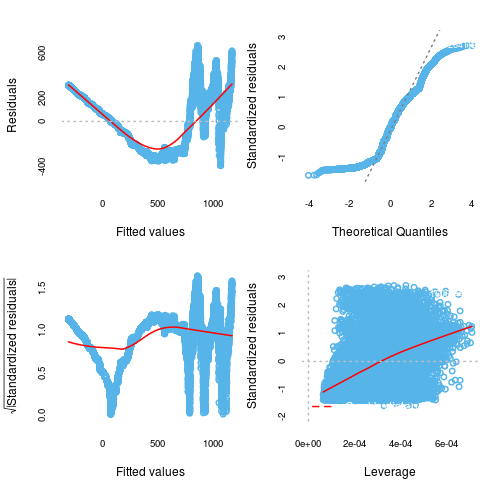References:

• Belsley, D. A., Kuh, E. and Welsch, R. E. (1980) Regression Diagnostics. New York: Wiley.
• Cook, R. D. and Weisberg, S. (1982) Residuals and Influence in Regression. London: Chapman and Hall.
• Firth, D. (1991) Generalized Linear Models. In Hinkley, D. V. and Reid, N. and Snell, E. J., eds: Pp. 55-82 in Statistical Theory and Modelling. In Honour of Sir David Cox, FRS. London: Chapman and Hall.
• Hinkley, D. V. (1975) On power transformations to symmetry. Biometrika 62, 101-111.
• McCullagh, P. and Nelder, J. A. (1989) Generalized Linear Models. London: Chapman and Hall.

## Predicted values

At last, let us compare the original data with the predicted values: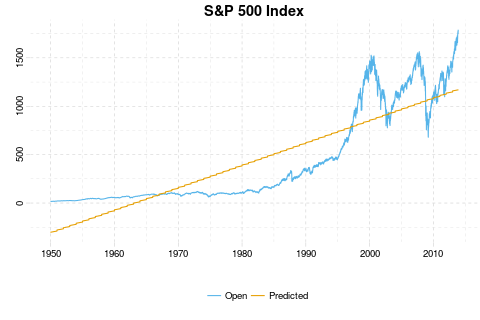References:

• Chambers, J. M. and Hastie, T. J. (1992) Statistical Models in S. Wadsworth & Brooks/Cole.

# ARIMA

Here we try to identify the best ARIMA model to better understand the data or to predict future points in the series. The model is chosen according to either AIC, AICc or BIC value is:

Damn, we could not fit a model:


We are terribly sorry, but this computational intensive process
is not allowed to be run on a time-series with more then 365 values.